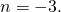Midterm 1 Preparation

# Midterm 1: Version E

1. Simplify the following:
1.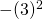2.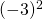3.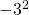4.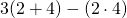5.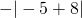1. Solve for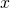in the equation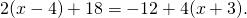2. Isolate the variable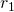in the equation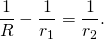3. Solve forin the equation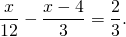4. Find the equation of the horizontal line that passes through the point5. Find the equation that has a slope of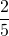and passes through the point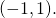6. Find the equation of the line passing through the points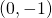and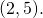7. Graph the relation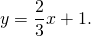For questions 9 to 11, find each solution set and graph it.

1.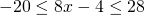2.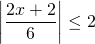3.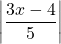>4. Graph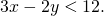5. Find three consecutive odd integers such that the sum of the first integer, two times the second integer, and three times the third integer is 94.
6. Karl is going to cut a 800 cm cable into 2 pieces. If the first piece is to be 3 times as long as the second piece, find the length of each piece.
7.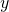varies jointly with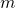and inversely with the square of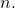If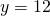when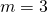and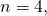find the constant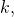then use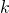to findwhenand